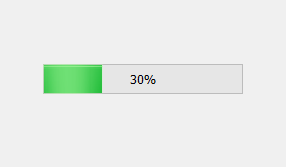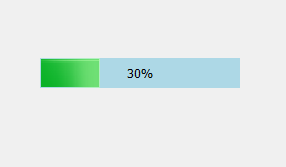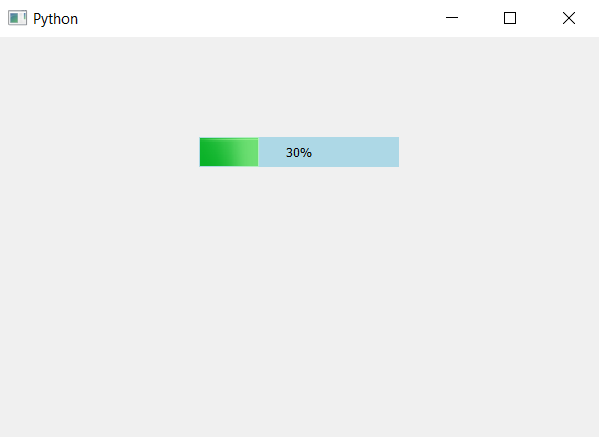Open In App

# PyQt5 – Background Color of Progress Bar

When we create a progress bar, by default there is no background color set to it, although the bar color is of green. In this article we will see how to set the background color to the progress bar. Below is the representation of normal progress bar vs the progress bar which has background color.In order to do this we have to change the CSS style sheet using `setStyleSheet` method, below is the style sheet.

```QProgressBar
{
background-color :lightblue;
border : 1px;
}
```

Below is the implementation.

 `# importing libraries``from` `PyQt5.QtWidgets ``import` `*` `from` `PyQt5 ``import` `QtCore, QtGui``from` `PyQt5.QtGui ``import` `*` `from` `PyQt5.QtCore ``import` `*` `import` `sys`` ` ` ` `class` `Window(QMainWindow):`` ` `    ``def` `__init__(``self``):``        ``super``().__init__()`` ` `        ``# setting title``        ``self``.setWindowTitle(``"Python "``)`` ` `        ``# setting geometry``        ``self``.setGeometry(``100``, ``100``, ``600``, ``400``)`` ` `        ``# calling method``        ``self``.UiComponents()`` ` `        ``# showing all the widgets``        ``self``.show()`` ` `    ``# method for widgets``    ``def` `UiComponents(``self``):``        ``# creating progress bar``        ``bar ``=` `QProgressBar(``self``)`` ` `        ``# setting geometry to progress bar``        ``bar.setGeometry(``200``, ``100``, ``200``, ``30``)`` ` `        ``# setting the value``        ``bar.setValue(``30``)`` ` `        ``# setting alignment to center``        ``bar.setAlignment(Qt.AlignCenter)`` ` `        ``# setting background color``        ``bar.setStyleSheet(``"QProgressBar"``                          ``"{"``                          ``"background-color : lightblue;"``                          ``"border : 1px"``                          ``"}"``)`` ` ` ` `App ``=` `QApplication(sys.argv)`` ` `# create the instance of our Window``window ``=` `Window()`` ` `# start the app``sys.exit(App.``exec``())`

Output :